Figures index

Arne Bergstrom

International Journal of Physics. 2014, 2(5), 146-150 doi:10.12691/ijp-2-5-3• Figure 1. Pareto distribution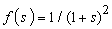compared to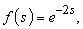coinciding for small s. Note the frequent occurrence of longer path lengths s in the Pareto distribution (cf Figure 2)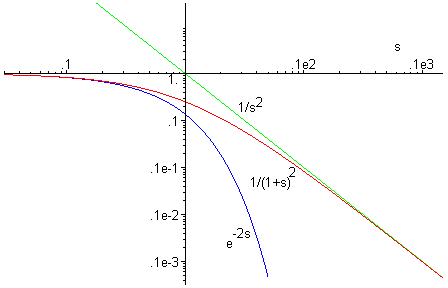• Figure 2. The Pareto distribution and negative exponential distribution in Figure 1 displayed in a loglog diagram in comparison with an inverse square distribution. The Pareto distribution agrees with a negative exponential for small s and with an inverse square law for large s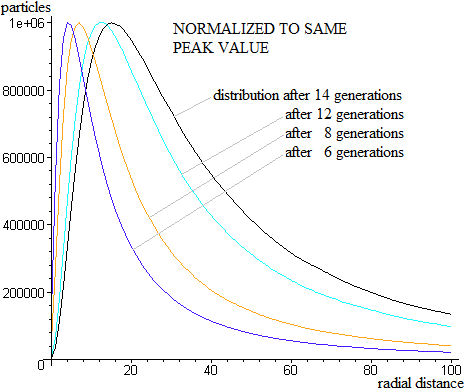• Figure 3A. Simulations of the particle distribution as function of radial distance for a large number of particles, all started at the origin and followed for 6, 8, 12, and 14 generations, respectively, of particle doubling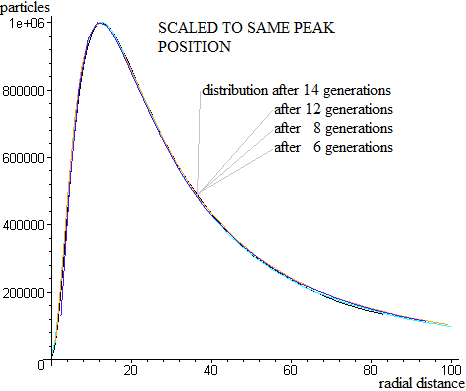• Figure 3B. The four curves in Figure 3A scaled to the same peak value and peak position, illustrating that the curves for different generations have the same functional form, and that the particle distribution thus is self-similar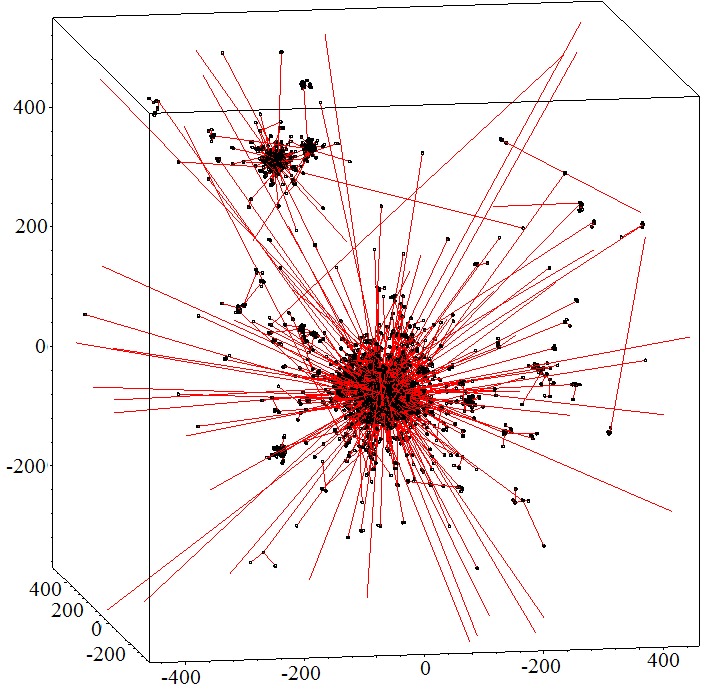• Figure 4A. Spatial distribution of collision points (black) and trajectories (red) for 14 generations of particle doubling after starting a particle at the origin. Note the many open trajectories (“dark energy”) of regime-II-trajectories leading to collision points far away outside the volume shown, and creating new clusters much further away. The closely-knit regime-I-trajectories in the dense clusters of collision points are interpreted as “dark matter”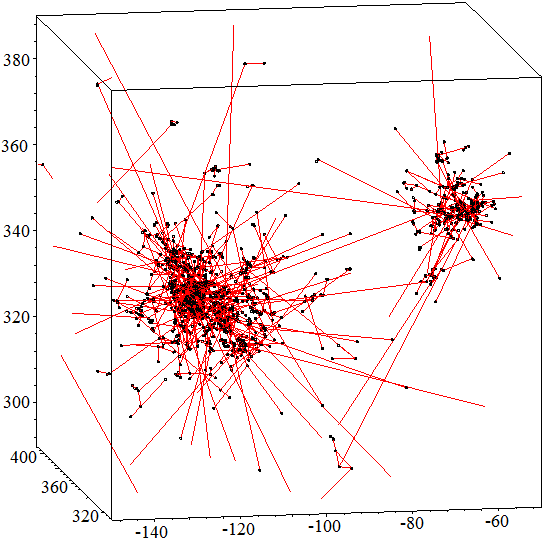• Figure 4B. Enlargement of the upper left corner of Figure 4A, showing a daughter cluster which in fact has just produced a second-generation daughter cluster to the right of the original daughter cluster